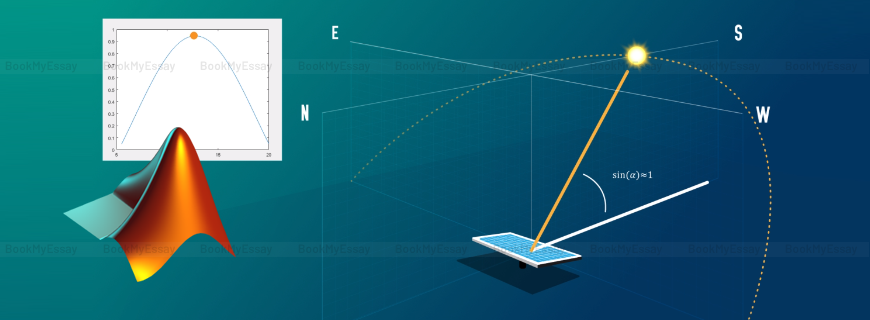# MATLAB Assignment HelpGet a Free Quote
It's free and always will be.
Page - +
words
NWL

## Online MATLAB Assignment Help

MATLAB or Matrix Laboratory is an extremely important high-performance technical computing language that smoothly integrates computing, programming and visualization. It creates an environment where mathematical solutions are made easy and interaction with computer for mathematical problems and solutions, computation and algorithmic development etc. become easier and faster for the students, researchers and experts.

Our experienced and well qualified MATLAB experts provides the premier online MATLAB assignment help service, which is very popular among the students of computer science, mathematics and technology etc.

### What is MATLAB and How Does it Work?

MATLAB is meant primarily for different numerical computing, the programming provides an optional toolbox that uses MuPAD symbolic engine which allows access to algebraic, geometrical and trigonometrical symbols for more intensive mathematical computing system. Later, the system has also added other facilities like: multi-domain simulation, and model based design system.

MATLAB is a fourth generation programming language which allows matrix operations, application of different functions, implementation of algorithms etc. Moreover, the dynamism of this programming language lies in the fact that it can smoothly create interfaces with other programming languages like C++, JAVA, Fortran, and Python etc.

There are millions of users of MATLAB around the world. In many higher level courses, students are given intensive knowledge on this programming language.

### Why Students Need MATLAB Programming Assignment Help?

Everyday hundreds of students from different parts of the world including the students studying computer science, mathematics and engineering in various reputed colleges and universities contact BookMyEssay for getting help for MATLAB programming assignment.

Assignments on Computer programming is hard to crack and when programming like MATLAB is concerned where intensive mathematical knowledge is mandatory, the situation worsens for the students. It is indeed tough to complete an assignment on MATLAB at very first attempt. Most of the students feel highly stressed and utterly dissatisfied with the assignments they have just completed. Hence, getting help with MATLAB assignment is the best option for the students.

Problems don’t stop there alone. Many other lacking often surface that severely hamper a student’s wish to complete assignments by their own effort. Shortage of time due too much engagement at classes, or at labs is another major hurdle for the students, while many other complains that they couldn’t understand the guidelines provided to them.  Actually there is no dearth of issues that keep on coming one after another that ultimately strangle a student’s wish to tackle MATLAB assignments by their own.

### Type of Assignments Commonly Given to the Students:

MATLAB basics constitute the elementary sides of linear algebra where various aspects of scientific operations such as: general algebraic calculation, mathematical modeling and analysis, simulation, etc. are integrated in one place. It is useful in several areas of science, and technology like computer science, statistics, physics, astronomy etc. Thus, MATLAB assignments can be given to the students from various fields and topics include –

• Computation and mathematical modeling.
• Testing of prototypes, simulation.
• Graphical analysis in science, engineering and technology.
• Construction of Graphical User Interfaces (GUIs) for the application of user-end applications.
• Synthesis and Expansion of Algorithms.
• Application and GUI Development.
• Data analysis, exploration, and visualization

### How MATLAB Experts Provide Support to the Students?

BookMyEssay has employed the well experienced MATLAB experts from different countries to deliver all necessary assistance to the students contacting the organization for MATLAB assignment writing help. These expert are vastly experienced in coding and applications. They also have all necessary know-how on how to complete MATLAB assignments, homework or essays right on time.

The MATLAB programmers take greatest care on coding to make them simple and high quality. Customized coding is done for each assignment even if two different assignments are same in nature. This eliminates any chance of plagiarism. All measures are taken by these experts to write the assignments by following the guidelines which is necessary to fetch maximum marks in assessments.

Some commonly solved topics of assignments often faced by these experts include the following –

• MATLAB image processing
• Mathematical modelling
• Image processing with MATLAB
• Root locus design using MATLAB
• MATLAB simulation project
• Automatic tracking algorithm using MATLAB

Actually, there is no dearth of topics on which such assignments can be given. But, experts associated with us knows how to solve these assignments successfully. Hence students relying of these professionals stay a step forward from their classmates as far as quality of assignments are concerned.

### Other Supporting Features:

Several other factors are also responsible for BookmyEassy’s extreme popularity –

• Students help desk remain open 24×7. Hence, students can contact whenever they feel they need help.
• Urgent online MATLAB assignment help is also available. Highly experience MATLAB programmers extend their helping service to the students who need completed assignments within 24 hours.
• Students are welcomed to resend their assignments for necessary changing and editing.
• The entire service is very much affordable to any student. Assignment Writing help on MATLAB programming from us is considered as the lowest one in the industry.